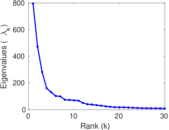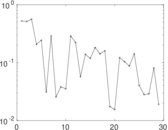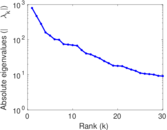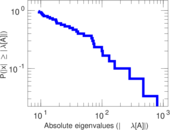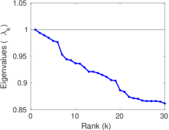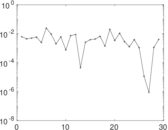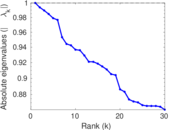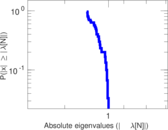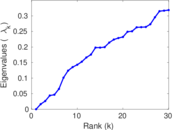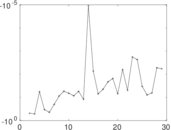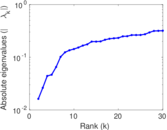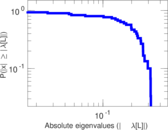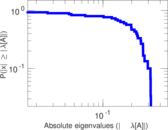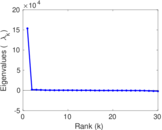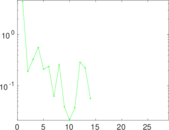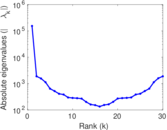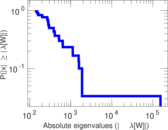# Wiktionary edits (uz)

This is the bipartite edit network of the Uzbek Wiktionary. It contains users and pages from the Uzbek Wiktionary, connected by edit events. Each edge represents an edit. The dataset includes the timestamp of each edit.

 Code `muz` Internal name `edit-uzwiktionary` Name Wiktionary edits (uz) Data source http://dumps.wikimedia.org/ AvailabilityDataset is available for download Consistency checkDataset passed all tests Category Authorship network Dataset timestamp 2017-10-20 Node meaning User, article Edge meaning Edit Network formatBipartite, undirected Edge typeUnweighted, multiple edges Temporal dataEdges are annotated with timestamps

## Statistics

 Size n = 153,595 Left size n1 = 306 Right size n2 = 153,289 Volume m = 497,719 Unique edge count m̿ = 315,106 Wedge count s = 12,363,094,229 Claw count z = 423,945,914,506,203 Cross count x = 1.174 × 1019 Square count q = 3,880,891,968 4-Tour count T4 = 80,500,143,180 Maximum degree dmax = 206,010 Maximum left degree d1max = 206,010 Maximum right degree d2max = 84 Average degree d = 6.480 93 Average left degree d1 = 1,626.53 Average right degree d2 = 3.246 93 Fill p = 0.006 717 76 Average edge multiplicity m̃ = 1.579 53 Size of LCC N = 146,647 Diameter δ = 16 50-Percentile effective diameter δ0.5 = 1.607 15 90-Percentile effective diameter δ0.9 = 3.472 11 Median distance δM = 2 Mean distance δm = 2.395 97 Gini coefficient G = 0.634 492 Balanced inequality ratio P = 0.277 254 Left balanced inequality ratio P1 = 0.014 920 1 Right balanced inequality ratio P2 = 0.406 304 Relative edge distribution entropy Her = 0.628 425 Power law exponent γ = 2.491 18 Tail power law exponent γt = 5.551 00 Tail power law exponent with p γ3 = 5.551 00 p-value p = 0.000 00 Left tail power law exponent with p γ3,1 = 1.581 00 Left p-value p1 = 0.001 000 00 Right tail power law exponent with p γ3,2 = 7.991 00 Right p-value p2 = 0.775 000 Degree assortativity ρ = −0.361 688 Degree assortativity p-value pρ = 0.000 00 Spectral norm α = 795.876 Algebraic connectivity a = 0.016 215 9 Spectral separation |λ1[A] / λ2[A]| = 1.687 97 Controllability C = 146,378 Relative controllability Cr = 0.995 999

## Plots

### Fruchterman–Reingold graph drawing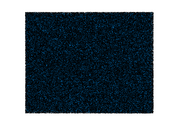### Degree distribution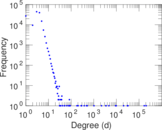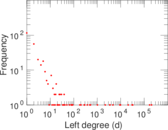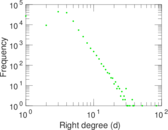### Cumulative degree distribution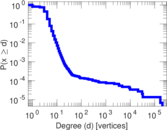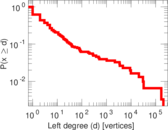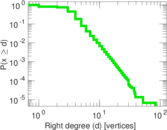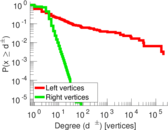### Lorenz curve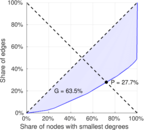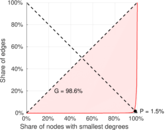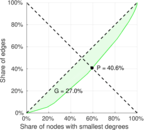### Spectral distribution of the adjacency matrix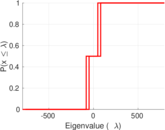### Spectral distribution of the normalized adjacency matrix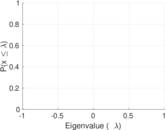### Spectral distribution of the Laplacian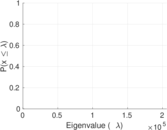### Spectral graph drawing based on the adjacency matrix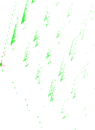### Spectral graph drawing based on the Laplacian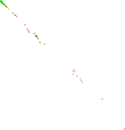### Spectral graph drawing based on the normalized adjacency matrix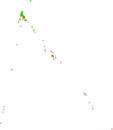### Degree assortativity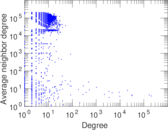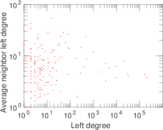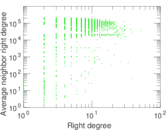### Zipf plot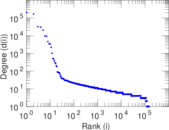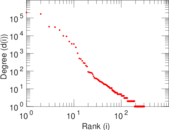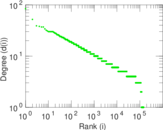### Hop distribution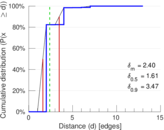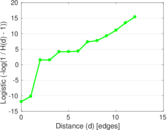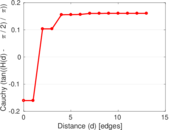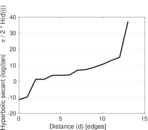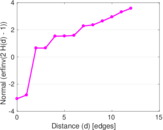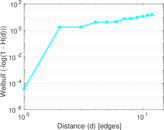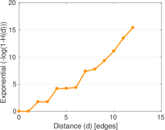### Delaunay graph drawing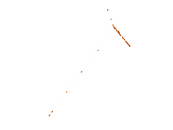### Edge weight/multiplicity distribution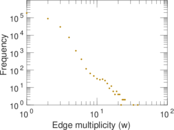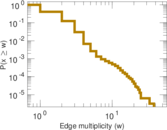### Temporal distribution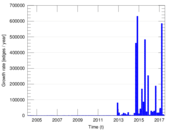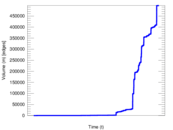### Diameter/density evolution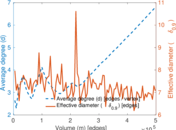### Matrix decompositions plots